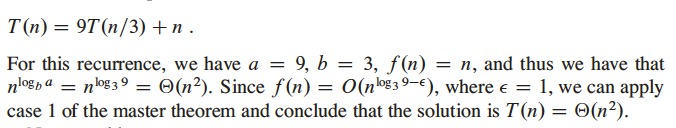July 17, 2019

# Divide and Conquer

• Merge sort is an example of a divide-and-conquer algorithm
• Recall the three steps (at each level) to solve a divide-and-conquer problem recursively
• Divide problem into subproblems
• Conquer problems by solving recursively
• Combine solutions to solve the original problem
• Recursive case: when the subproblems are large enough to be solved recursively
• Base case: when subproblems are small enough that we don’t need to use recursion any more, we say that the recursion bottoms out

# Recurrences

• Recurrences are essential for the divide-and-conquer paradigm
• Give a natural way to characterize the running times of divide-and-conquer algorithms
• Recurrence
• An equation or inequality
• Describes a function in terms of its value on smaller inputs
• Worst case running time for MERGE-SORT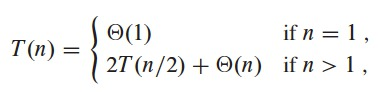• This recurrence has solution: $$T(n) = \Theta(n \lg n)$$.

# Recurrences

• There are many forms of recurrences
• A recursive algorithm dividing subproblems into unequal sizes, $$2/3$$ to $$1/3$$ split, with divide and combine times linear
• $$T(n) = T(2n/3) + T(n/3) + \Theta(n)$$.
• Linear search, each subproblem has one element less than the original one
• $$T(n) = T(n - 1) + \Theta(1)$$.

# Recurrences

• Three methods for solving recurrences, for obtaining asymptotic $$\Theta$$ or $$O$$ bounds on the solution
• Substitution method
• We guess a bound
• Then use mathematical induction to prove our guess correct.

# Recurrences

• Three methods for solving recurrences, for obtaining asymptotic $$\Theta$$ or $$O$$ bounds on the solution
• Recursion-tree method
• Converts the recurrence into a tree
• Nodes represent costs incurred at levels of recursion
• Use techniques for bounding summations and solve the recurrence

# Recurrences

• Three methods for solving recurrences, for obtaining asymptotic $$\Theta$$ or $$O$$ bounds on the solution
• Master method
• Provides bounds for recurrences of the form $$T(n) = aT(n/b) + f(n)$$
• $$a \geq 1$$, $$b > 1$$, $$f(n)$$ is a given function
• This is a recurrence that solves a problem that was divided into $$a$$ subproblems, each subproblem is $$1/b$$ of the original size, and divide and combine takes $$f(n)$$ time
• Has 3 cases, need to memorize

# Recurrences

• Sometimes we will use recurrences that are inequalities
• $$T(n) \leq 2T(n/2) + \Theta(n)$$, to get an upper bound on $$T(n)$$, with $$O$$-notation
• $$T(n) \geq 2T(n/2) + \Theta(n)$$, to get a lower bound on $$T(n)$$, with $$\Omega$$-notation

# Technicalities in Recurrences

• Some technical details when we state and solve recurrences
• In a call to MERGE-SORT with $$n$$ elements, when $$n$$ is odd we use subproblems of size $$\lfloor n/2 \rfloor$$ and $$\lceil n/2 \rceil$$, we ignore this and treat it as if $$n$$ were always even
• Boundary conditions are usually ignored
• i.e. algorithms for sufficiently small $$n$$ have $$T(n) = \Theta(1)$$, then we omit statements on boundary conditions
• We often omit floors, ceilings, and boundary conditions

# The Maximum-subarray Problem

• Invest in the Volatile Chemical Corporation
• Stock price is volatile
• Allowed to buy one unit of stock only one time and then sell it at a later date
• Allowed to know what the price of the stock will be in the future
• Goal: maximize profit

# The Maximum-subarray Problem

• Lowest price at day 7
• Buy at lowest price and sell at highest price? NOT always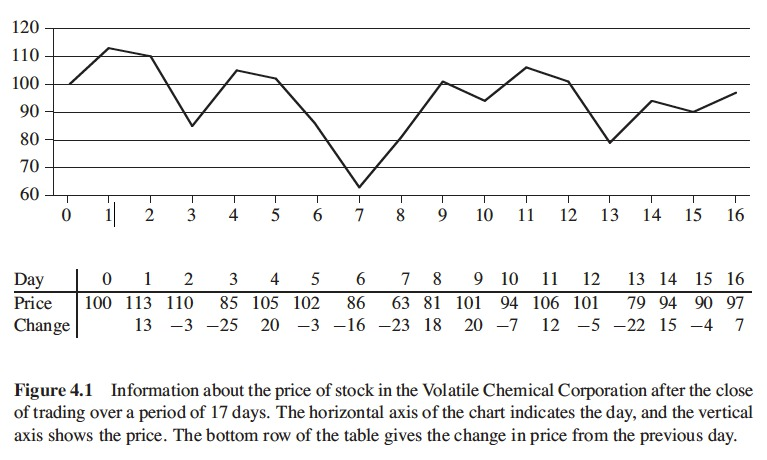# The Maximum-subarray Problem

• Highest profit: buy at 7 (day 2) and sell at 10 (day 3)
• Day 2 is not the one with the lowest price, nor day 3 is the one with the highest price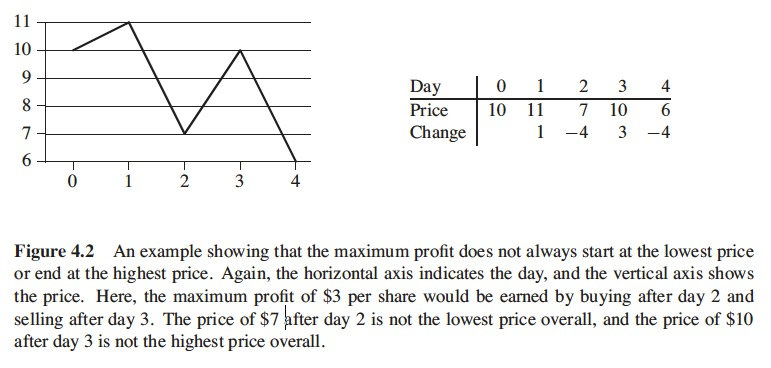# A Brute-force Solution

• Try every possible pair of buy and sell dates (buy precedes sell date)
• Period of $$n$$ days has $$\binom {n}{2}$$ pairs of dates
• $$\binom {n}{2}$$ is $$\Theta(n^2)$$
• Best we could hope is to evaluate each pair in constant time and approach would take $$\Omega(n^2)$$
• Can we do better?

# A Transformation

• Look at the problem in a different way
• Find a sequence of days in which the net change from the first day to the last one is maximum
• Treat the daily changes as an array A
• Now we look for the nonempty, contiguous subarray of A whose values have the largest sum, the maximum subarray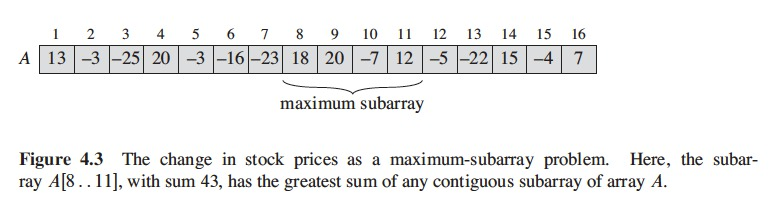# A Transformation

• Maximum subarray of $$A[1..16]$$ is $$A[8..11]$$ with sum 43
• Buy just before day 8 (after day 7) and sell after day 11, earning profit of \$43 per share
• Need to check $$\binom {n-1}{2} = \Theta(n^2)$$ subarrays for a period of $$n$$ days
• Bruteforce solution still takes $$\Theta(n^2)$$

# A Solution Using Divide-and-conquer

• We have an array $$A[low .. high]$$
• In divide and conquer we divide the subarray into 2 subarrays of equal size as possible
• Find midpoint of the subarray, and get 2 subarrays $$A[low .. mid]$$ and $$A[mid+1 .. high]$$
• But any contiguous subarray $$A[i .. j]$$ of $$A[low .. high]$$ must fall in:
• Entirely in subarray $$A[low .. mid]$$, $$low \leq i \leq j \leq mid$$
• Entirely in subarray $$A[mid+1 .. high]$$, $$mid < i \leq j \leq high$$
• Crossing the midpoint, $$low \leq i \leq mid < j \leq high$$.
• A maximum subarray of $$A[low .. high]$$ must have the greatest sum over all the three cases stated before
• We can find subarrays of $$A[low .. mid]$$ and $$A[mid+1 .. high]$$ recursively
• Not for subarrays crossing the midpoint
• We do it without recursion

# A Solution Using Divide-and-conquer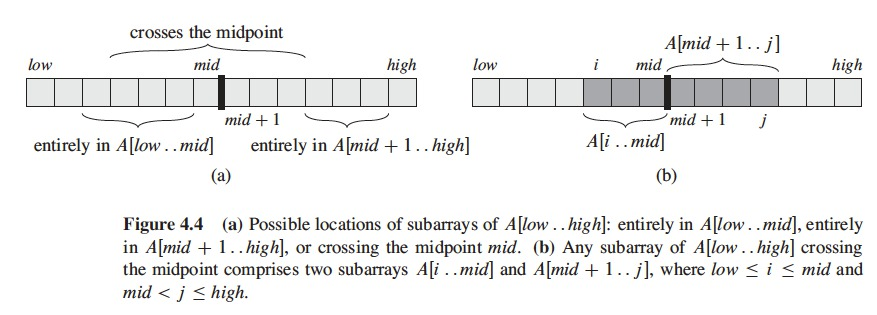# A Solution Using Divide-and-conquer

• How does this procedure work?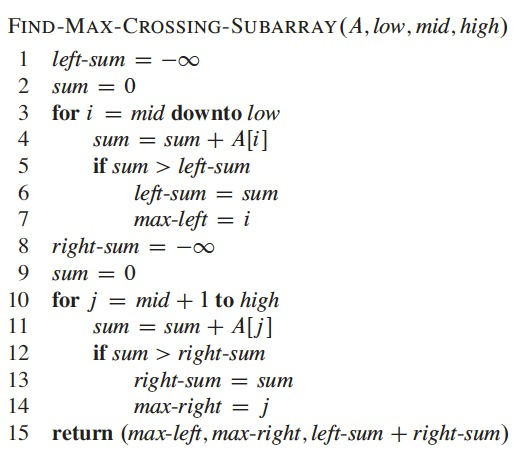• What is its running time?
• Number of iterations: $$(mid - low + 1) + (high - mid) = high - low + 1 = n$$

# A Solution Using Divide-and-conquer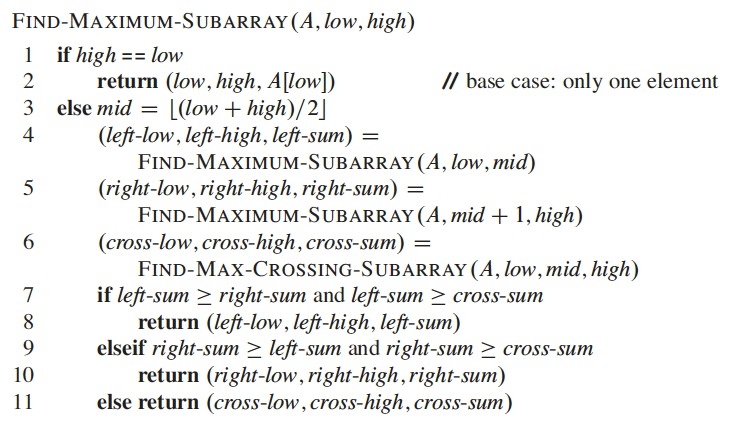# A Solution Using Divide-and-conquer

• Lines 1,2 is the base case
• Lines 3-11 handle recurssive case
• Line 3 does the divide part, computes mid
• Left subarray $$A[low .. mid]$$, right subarray $$A[mid+1 .. high]$$
• Lines 4 and 5 do the conquer by recursion
• Lines 6 to 11 are the combine part
• Line 6 finds max subarray crossing the midpoint
• Line 7 tests if left subarray contains max sum
• Line 9 tests if right subarray contains max sum
• Line 11 returns max subarray if crosses midpoint

# Analyzing the Divide-and-conquer Algorithm

• Which recurrence describes the running time of the FIND-MAXIMUM-SUBARRAY procedure?
• Assumption: the original problem size is a power of 2, all subproblem sizes are integers
• $$T(n)$$, denotes running time of FIND-MAXIMUM-SUBARRAY on subarray of size $$n$$
• Line 1, constant time, line 2, constant time, line 3, constant time
• Base case $$T(1) = \Theta(1)$$.

# A Solution Using Divide-and-conquer

• Subproblems in recursive part (lines 4, 5) work on subarray of size $$n/2$$
• We spend $$T(n/2)$$ time solving each, we solve 2 of them
• We spend $$2T(n/2)$$
• FIND-MAX-CROSSING-SUBARRAY takes $$\Theta(n)$$
• Lines 7-11 take $$\Theta(1)$$ time
• Then, for the recursive case we have:
• $$T(n) = \Theta(1) + 2T(n/2) + \Theta(n) + \Theta(1)$$
• $$= 2T(n/2) + \Theta(n)$$.

# A Solution Using Divide-and-conquer

• Combining base case and recursive case we have the recurrence: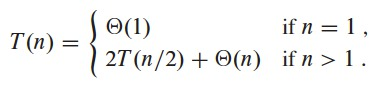• The solution to this recurrence is: $$T(n) = \Theta(n \lg n)$$.

# The Substitution Method

• Now we need to solve recurrences
• Substitution method
• 2 steps
1. Guess the form of the solution
2. Use mathematical induction to find the constants and show that the solution works
• Powerful method
• Only applies in cases that the answer is easy to guess
• To establish upper or lower bounds of a recurrence

# The Substitution Method: Example

• For the recurrence $$T(n) = 2T(\lfloor n/2 \rfloor) + n$$.
• We guess a solution, upper bound $$T(n) = O(n \lg n)$$
• By definition of $$O$$ we need to prove that $$T(n) \leq cn\lg n$$ for appropriate constant $$c > 0$$
• We assume this holds for $$m < n$$, in particular for $$m = \lfloor n/2 \rfloor$$
• This yields $$T(\lfloor n/2 \rfloor) \leq c \lfloor n/2 \rfloor \lg(\lfloor n/2 \rfloor)$$.
• Now we substitute in the recurrence
• Recurrence: $$T(n) = 2T(\lfloor n/2 \rfloor) + n$$
• Substitute $$T(n)$$ by $$T(n/2)$$ in our guess
• Now we have: $$T(n) \leq 2(c\lfloor n/2 \rfloor \lg ( \lfloor n/2 \rfloor)) + n$$

# The Substitution Method: Example

• We substitute into the recurrence: $$T(n) \leq 2(c\lfloor n/2 \rfloor \lg ( \lfloor n/2 \rfloor)) + n$$
• $$\leq cn \lg (n/2) + n$$
• $$= cn \lg n - cn \lg 2 + n$$
• $$= cn \lg n - cn + n$$
• $$\leq cn \lg n$$
• the last step holds if $$c \geq 1$$.

# The Substitution Method: Example

• We still need to prove that this solution is true for boundary conditions
• We do this by showing that boundary conditions are suitable for base cases of the inductive proof
• Choose constant $$c$$ large enough for $$T(n) \leq cn \lg n$$ works for boundary conditions
• For asymptotic notation we require to prove $$T(n) \leq cn \lg n$$ for $$n \geq n_0$$, we choose $$n_0$$

# The Substitution Method: Example

• We avoid difficult boundary condition for $$T(1) = 1$$ for the induction test (not the recurrence)
• In the inductive proof with $$n = 1$$, $$T(1) \leq c1 \lg 1 = 0$$, then it doesn’t correspond with $$T(1) = 1$$, and base case fails.
• But we need to prove $$T(n) \leq cn \lg n$$ for $$n \geq n_0$$
• And we are free to choose $$n_0$$
• We remove the troublesome boundary by removing from the recurrence by seting $$n_0 > 3$$ and in the inductive proof with $$n > 1$$
• And we replace $$T(1)$$ by $$T(2)$$ and $$T(3)$$ as base cases of the inductive proof
• We make the base cases $$T(2)$$ and $$T(3)$$ instead of $$T(1)$$
• Derive from the recurrence
• Recurrence: $$T(n) = 2T(\lfloor n/2 \rfloor) + n$$, given that $$T(1) = 1$$
• $$T(2) = 4$$
• $$T(3) = 5$$
• $$T(2) \leq c2 \lg 2 = 2c$$, $$c \geq 2$$
• $$T(3) \leq c3 \lg 3 = 4.75c$$, $$c \geq 2$$

# Making a Good Guess

• There is no general way to guess correct solutions to recurrences
• Requires experience and creativity
• Some heuristics
• Use recursion trees to generate good initial values
• If a recurrence is similar to another one, use a similar solution

# The Recursion-tree Method

• Visualize the iteration of a recurrence
• Draw a recursion tree and obtain a good initial solution
• We use the substitution method to proof
• Recursion tree
• Each node represents the cost of a subproblem in the set of calls to recursive functions
• We sum costs per level and determine the total cost of all levels of recursion
• Useful when the recurrence describes execution time of a divide-and-conquer algorithm

# The Recursion-tree Method

• To solve recurrence $$T(n) = 3T(\lfloor n/4 \rfloor) + \Theta(n^2)$$
• We create the recursion tree for $$T(n) = 3T(n/4) + cn^2$$
• We include the coefficient $$c > 0$$
• We assume that $$n$$ is an exact power of 4

# The Recursion-tree Method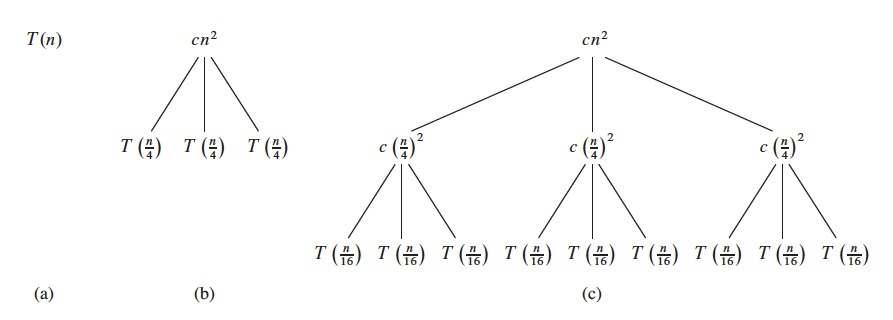# The Recursion-tree Method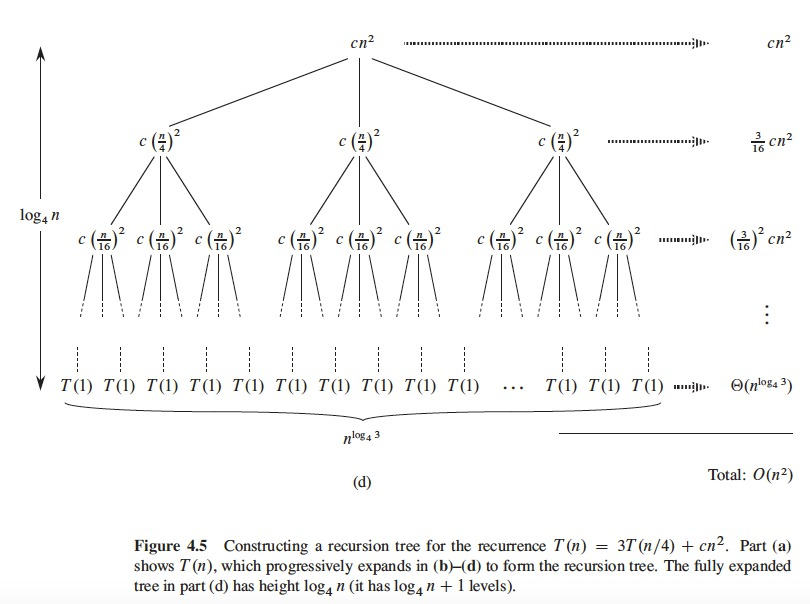# The Recursion-tree Method

• The size of the problem decreases with the depth of the tree
• Eventually, we reach the boundary condition
• How far from the root do we get?
• The size of the subproblem for a node at depth $$i$$ is $$n/4^i$$
• The size gets to $$n = 1$$ when $$n/4^i = 1$$, or $$i = \log_4 n$$
• Then, the tree has $$\log_4 n+1$$ levels $$0, 1, 2, \dots, \log_4 n)$$

# The Recursion-tree Method

• After we determine the cost for each level of the tree
• Each level has 3 times more nodes than the previous level
• The number of nodes at depth $$i$$ is $$3^i$$
• Each node at depth $$i$$ for $$i = 0,1,2, \dots, \log_4 n-1$$ has a cost of $$c(n/4^i)^2$$
• Multiplying, we see that the cost of all nodes at depth $$i$$ for $$i = 0, 1, 2, \dots \log_4 n-1$$ is $$3^i c(n/4^i)^2 = (3/16)^i cn^2$$
• The last level at depth $$log_4 n$$ has $$3^{\log_4 n} = n^{log_4 3}$$ nodes, each with a cost of $$T(1)$$ with a total cost of $$n^{log_4 3}T(1)$$ with $$\Theta(n^{log_4 3})$$.

# The Recursion-tree Method

• We sum the costs of all levels to get the cost of all the tree
• $$T(n) =$$
• $$cn^2 + \frac{3}{16}cn^2 + (\frac{3}{16})^2 cn^2 + \dots + (\frac{3}{16})^{log_4 n-1} cn^2 + \Theta(n^{log_4 3})$$
• $$= \sum_{i=0}^{log_4 n-1} (\frac{3}{16})^i cn^2 + \Theta(n^{log_4 3})$$
• $$= \frac{(3/16)^{log_4 n} - 1}{(3/16) - 1} cn^2 + \Theta(n^{log_4 3})$$.

# The Recursion-tree Method

• We can use an infinite and decreasing geometric serie as upper bound, equation A.6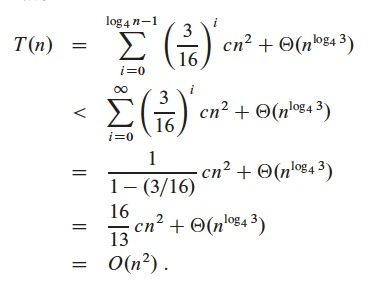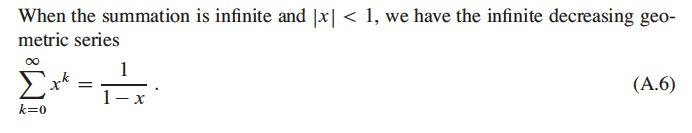# The Master Method

• Master method provides cookbook method for recurrences of the form
• $$T(n) = aT(n/b) + f(n)$$
• where $$a \geq 1$$ and $$b>1$$ are constants
• $$f(n)$$ is asymptotically positive
• The recurrence describes the execution time of an algorithm that
• Divides a problem of size $$n$$ into $$a$$ subproblems
• Each subproblem of size $$n/b$$
• $$a$$ and $$b$$ are positive constants
• The $$a$$ subproblems are solved recursively
• In time $$T(n/b)$$
• The cost of dividing the problem and combining the results is given by $$f(n)$$

# The Master Method

• The master theorem
• Given $$a \geq 1$$ and $$b>1$$ constants, given $$f(n)$$ a function
• Given $$T(n)$$ defined by non-negative integers by recurrence
• $$T(n) = aT(n/b) + f(n)$$
• $$n/b$$ can be $$\lfloor n/b \rfloor$$ or $$\lceil n/b \rceil$$, then $$T(n)$$ has the following asymptotic bounds as: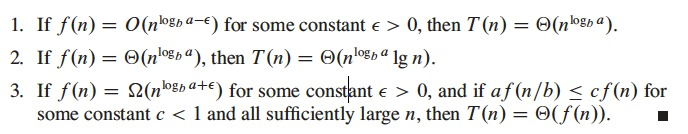# The Master Method

• In all cases we compare $$f(n)$$ with $$n^{log_b a}$$
• The solution to the recurrence is dominated by the largest of the 2 functions
• Case 1: $$n^{log_b a}$$ is the largest, the solution is $$T(n) = \Theta(n^{log_b a})$$
• Case 3: $$f(n)$$ is larger, the solution is $$T(n) = \Theta(f(n))$$
• Case 2: the two functions are of the same size, we multiply for a logarithmic factor, $$T(n) = \Theta(n^{log_b a} \lg n) = \Theta(f(n) \lg n)$$

# The Master Method

• Some technical aspects
• In case 1:
• $$f(n)$$ must be polynomially smaller than $$n^{log_b a}$$
• Asymptotically smaller than $$n^{log_b a}$$ by a factor of $$n^{\epsilon}$$ for a constant $$\epsilon > 0$$
• In case 3:
• $$f(n)$$ must be polynomially larger than $$n^{log_b a}$$
• It should also satisfy the regularity condition that $$af(n/b) \leq cf(n)$$
• This condition is satisfied by most functions polynomially bounded that we can find
• $$\rightarrow$$ the 3 cases don’t cover all possibilities of $$f(n)$$

# The Master Method